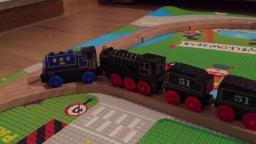# Expressway 5837

The expressway covers the distance from the starting point to the final station in 4 hours and 20 minutes. A passenger train, whose average speed is 30 km/h less, will cover this distance in 7 h 40 min. What is the speed of the express train, and what is the speed of the passenger train?

a =  69 km/h
b =  39 km/h

### Step-by-step explanation:

a·(4+20/60) = b·(7+40/60)
b = a - 30

260a-460b = 0
a-b = 30

Row 2 - 1/260 · Row 1 → Row 2
260a-460b = 0
0.77b = 30

b = 30/0.76923077 = 39
a = 0+460b/260 = 0+460 · 39/260 = 69

a = 69
b = 39

Our linear equations calculator calculates it.Did you find an error or inaccuracy? Feel free to write us. Thank you!

Tips for related online calculators
Do you have a system of equations and looking for calculator system of linear equations?
Do you want to convert velocity (speed) units?
Do you want to convert time units like minutes to seconds?